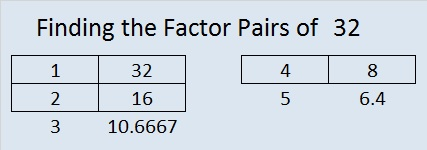# 32 and Hooked on Factoring

32 is a composite number. 32 = 1 x 32, 2 x 16, or 4 x 8.  Factors of 32: 1, 2, 4, 8, 16, 32. Prime factorization: 32 = 2 x 2 x 2 x 2 x 2, which can also be written 32 = 2⁵.When 32 is a clue in the FIND THE FACTORS puzzles, always use 4 and 8 as the factors.Here is a hook-shaped FIND THE FACTORS 1-10 puzzle, and YOU can solve in by hook or by crook!

The puzzle has a few square numbers: 16, 4, 81, 36, 36, 9, 1, and 25. Several square numbers can only be factored one way in a FIND THE FACTORS puzzle, while others can be factored 2 or 3 ways.

Obviously, 6 x 6 = 36 will not let us off the hook both times that 36 appears. One of those times we must use 4 x 9 = 36. This puzzle reminds us that 36 is sometimes a square and sometimes a rectangle.

The 16 isn’t a square in this puzzle either. Sometimes 4 and 9 are rectangles, but not in this case.

81 and 25 are always square in the FIND THE FACTORS puzzles because even though 1 and 3 are allowed to be factors, 25, 27, and 81 are greater than 10 and not allowed as possible factors to solve the puzzle.

To solve this puzzle, write the numbers from 1 to 10 in the top row and again in the first column so that those numbers are the factors of the given clues. Don’t fall for those slightly tricky clues hook, line, and sinker!

This week’s puzzles are also available in an excel file here. If you have a spreadsheet program on your computer, you can access it. If you enable editing in excel, you can type your answers directly onto the puzzle, and you can also easily print the puzzles. I’m hooked on factoring. I hope you’ll get hooked, too!

Here are all the factors to last week’s level 2 FIND THE FACTORS 1-12 snowflake puzzle:## One thought on “32 and Hooked on Factoring”

This site uses Akismet to reduce spam. Learn how your comment data is processed.